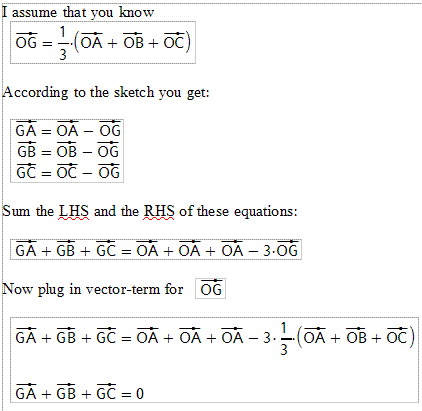# Vector Proof Centroid Theorem

I am trying to figure out how to prove the 2:1 ratio of a triangle's medians at the centroid using vectors. Example if I had a triangle ABC with midpoints D of BC, E of AC and F of AB. I know G is where the medians intersect. I have seen many proofs and understand the process that proves the addition of the vectors from the centroid to the vertices are zero i.e. GA+GB+GC=0.Does this prove the 2:1 ratio? I cannot find anything explaining how to prove the actual 2:1 ratio. I am not sure if I am missing something or what. Any help or suggestions would be appreciated. Thanks.

tiny-tim
Homework Helper
I am trying to figure out how to prove the 2:1 ratio of a triangle's medians at the centroid using vectors. Example if I had a triangle ABC with midpoints D of BC, E of AC and F of AB. I know G is where the medians intersect. I have seen many proofs and understand the process that proves the addition of the vectors from the centroid to the vertices are zero i.e. GA+GB+GC=0.
View attachment 14402
Does this prove the 2:1 ratio? I cannot find anything explaining how to prove the actual 2:1 ratio. I am not sure if I am missing something or what. Any help or suggestions would be appreciated. Thanks.

Hi pjallen58!Yes, because, for example, a + 2(d) is 1/3 of the way,

and that's a + 2(1/2(b + c)), = a + b + c!